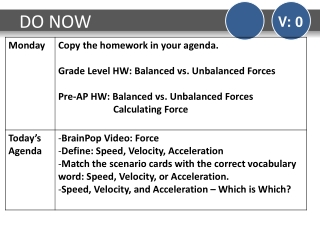DownloadDownload PresentationDO NOW

# DO NOW

Télécharger la présentation## DO NOW

- - - - - - - - - - - - - - - - - - - - - - - - - - - E N D - - - - - - - - - - - - - - - - - - - - - - - - - - -
##### Presentation Transcript

1. DO NOW V: 0

2. TEKS

3. BrainPop Video V: 0 BrainPop: Force

4. Speed, Velocity, Acceleration V: 0 Add notebook page for Speed, Velocity, and Acceleration.

5. Speed, Velocity, Acceleration V: 2 With your shoulder partner, match the scenario cards with the correct vocabulary word: Speed, Velocity, or Acceleration. Select 2 examples for each for speed, velocity and acceleration (or come up with your own) and copy the examples into your notebook Complete “Which is Which?” (RM9) – Exit Ticket

6. DO NOW V: 0

7. DO NOW V: 0

8. Review Chemistry Unit Exam V: 2 Review the Chemistry Unit Exam

9. TEKS

10. Force Foldable V: 0 Create a FORCE foldable by taping/gluing the handouts in your INB.

11. Force Foldable V: 0 What is force?

12. Force Foldable V: 0 What is force? A push or pull that can change the motion of an object.

13. Force Foldable V: 0 What 2 things does every force have?

14. Force Foldable V: 0 What 2 things does every force have? Force has a strength and direction.

15. Force Foldable V: 0 How is force measured?

16. Force Foldable V: 0 How is force measured? Force is measured in Newtons.

17. Force Foldable V: 0 Describe and give examples of balanced and unbalanced forces.

18. Net Force Practice Problems V: 0 When the arrows are pointing in the same direction…

19. Net Force Practice Problems V: 0 When the arrows are pointing in the same direction… ADD

20. Net Force Practice Problems V: 0 When the arrows are pointing in the same direction… ADD When the arrows are pointing in opposite directions…

21. Net Force Practice Problems V: 0 When the arrows are pointing in the same direction… ADD When the arrows are pointing in opposite directions… SUBTRACT

22. Net Force Practice Problems V: 0 Copy each of the practice problems in your INB and then answer the three questions. What is the net force? Are the forces balanced or unbalanced? Describe the motion of the object. (None, Accelerates Right, Accelerates Left) 10 N 20 N

23. Net Force Practice Problems V: 0 Copy each of the practice problems in your INB and then answer the three questions. What is the net force? Are the forces balanced or unbalanced? Describe the motion of the object. (None, Accelerates Right, Accelerates Left) 30 N 30 N

24. Net Force Practice Problems V: 0 Copy each of the practice problems in your INB and then answer the three questions. What is the net force? Are the forces balanced or unbalanced? Describe the motion of the object. (None, Accelerates Right, Accelerates Left) 20 N 50 N

25. Net Force Practice Problems V: 0 Copy each of the practice problems in your INB and then answer the three questions. What is the net force? Are the forces balanced or unbalanced? Describe the motion of the object. (None, Accelerates Right, Accelerates Left) 40 N 40 N

26. Net Force Practice Problems V: 0 Copy each of the practice problems in your INB and then answer the three questions. What is the net force? Are the forces balanced or unbalanced? Describe the motion of the object. (None, Accelerates Right, Accelerates Left) 30 N 15 N

27. Exit Ticket V: 0 Complete the Exit Ticket

28. DO NOW V: 0 25 N 15 N

29. TEKS

30. Tug-of-War V: 0 Create the table below in your INB.

31. Tug-of-War Simulator V: 0 Simulator Click on “Run Now”

32. Tug-of-War V: 0 Describe an example where there was an unbalanced force. How did you know it was unbalanced?

33. Tug-of-War V: 0 Draw a picture of a trial where the forces were unbalanced with arrows showing the direction of the forces.

34. Tug-of-War V: 0 Draw a picture of a trial where the forces were balanced with arrows showing the direction of the forces.

35. Reading & Cornell Notes V: 0 Read: Force and Motion Cornell Notes